# Heat and Mass Transfer along Vertical Channel in Porous Medium with Radiation Effect and Slip Condition

Heat and Mass Transfer along Vertical Channel in Porous Medium with Radiation Effect and Slip Condition

Halima UsmanFazle Mabood Giulio Lorenzini

Department of Mathematics, Usmanu Danfodiyo University, Sokoto, Nigeria

Department of Mathematics, University of Peshawar, Pakistan

Department of Industrial Engineering, University of Parma, 43124 Parma Italy

Corresponding Author Email:
hhalimausman@yahoo.com
Page:
129-136
|
DOI:
https://doi.org/10.18280/ijht.340119
|
Accepted:
|
Published:
31 March 2016
| Citation

OPEN ACCESS

Abstract:

This paper deals with the numerical investigation of simultaneous heat and mass transfer flow of a viscous, incompressible and radiating fluid along a vertical channel in a porous medium. The non-linear equations governing the flow for heat and mass transfer are solved by using a very efficient numerical scheme of Runge-Kutta Fehlberg fourth fifth (RK5-45) order method. The values of velocity, temperature, concentration, skin friction coefficient, rate of heat and mass transfer are found for different parameters involved in the formulation via magnetic, suction and slip parameters. It was found that the magnetic field and suction parameters have prominent effects on fluid flow, heat and mass transfer characteristics for the considered configuration.

Keywords:

Convection, Heat transfer, Mass transfer, MHD, Porosity.

1. Introduction

The important of heat and mass transfer occurs as a result of combined buoyancy effects of temperature and concentration differences through chemical species, which plays an important role in chemical engineering, aeronautics and geophysics. Some industrial applications are found in polymer production, food processing and drying as well as manufacturing of ceramics. Nield and Bejan  and Ingham and Pop  made comprehensive reviews of the studies of heat and mass transfer in relation to the above applications.

In the history of fluid flow through channels, Navier investigated a boundary condition of the fluid slip at a solid surface such that the velocity of the solid surface is proportional to the shear stress at the surface, that is $u=h \frac{\partial u}{\partial y}$, where $h$ is the slip coefficient and $u$ is the velocity along the $x$- axis Goldstein . The flow regime is called the slip flow regime and it is effects cannot be neglected due to it is importance in science, technology and industrialization, such as it is application in micro-channels and lubrication of mechanical device where a thin film of lubricant is attached to the surface slipping over one another or when the surfaces are coated with special coating in order to reduce the friction between them. The slip condition has been used in studies of fluid flow by many researchers. Bhattacharyya et al.  analyzed slip effects on boundary layer stagnation point flow and heat transfer towards a shrinking sheet. Important work on the effect of slip boundary condition on the flow of Newtonian fluid due to a stretching sheet was reported by Anderson  whilst Hayat et al.  considered MHD flow and heat transfer characteristics for the boundary layer flow over a permeable stretching sheet with thermal slip conditions. In another article, Aziz  investigated the boundary layer slip flow over a flat plate with constant heat flux condition at the surface. Similarly, Mohammed and Suneetha  discussed the effects of thermal diffusion and chemical reaction on MHD transient free convection flow past a porous vertical plate with radiation, temperature gradient heat source in slip flow regime. Krishnendu  presented a mathematical model to analyze the steady boundary layer slip flow and mass transfer with nth order chemical reaction past a porous plate embedded in a Darcy porous medium. Chand et al.  studied the combined effect of slip and jump boundary condition viscoelastic fluid with Soret effects. The theoretical analysis of unsteady hydrodynamic free convective flow of a viscoelastic fluid past an infinite vertical porous channel through a porous medium was recently, investigated by Aarti and Gorla . Most recently, Nityananda and Rajendra  examined the effect of slip condition on unsteady MHD oscillatory flow in a channel filled with saturated porous medium in the presence of transverse magnetic field and radiative heat and mass transfer. Additionally, Gbadeyan and Dada  investigated radiation and heat transfer effects on MHD non-Newtonian unsteady flow in a porous medium with slip condition. Ahmed and Kishore  reported MHD mass transfer flow past a vertical porous plate embedded in a porous medium in a slip flow regime with thermal radiation and chemical reaction. Ziya Uddin and Manoj  studied radiation effect on unsteady MHD heat and mass transfer flow on a moving inclined porous heated plate in the presence of chemical reaction, whilst Loganathan and Sivapoornapriya  studied radiation and chemical reaction effects on unsteady natural convection flow of a micropolar fluid past a vertical moving porous plate. Inspite of all these studies, it is observed that radiative transport is often comparable, and hence associated with that of convective heat and mass transfer in several practical applications. Therefore it is of great significance to the researchers to study heat and mass transfer with radiation effect and slip condition.

2. Mathematical Formulation

Consider a one-dimensional unsteady MHD laminar boundary layer flow of a viscous, incompressible and radiating fluid along a vertical channel in a porous medium. A uniform transverse magnetic field $B_{0}$is taken to be acting along the $y^{\prime}$- axis. The $x^{\prime}$- axis is taken along the plate in the vertically upward direction and $y^{\prime}$- axis is perpendicular to the wall of the plate in the direction of the applied uniform magnetic field (see fig. 1). The fluid has constant viscosity, constant thermal conductivity and constant chemical reaction. It is assumed that the viscous dissipation is negligible and there is no applied voltage, which implies the absence of an electric field.

## figure_1.png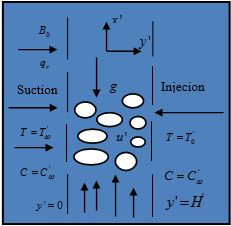Figure 1. Physical configuration of the problem

The Rosseland approximation is used to describe the radiative heat flux. By the above assumptions, the governing equations of the flow are given by:

$\frac{\partial v^{\prime}}{\partial y^{\prime}}=0$(1)

$v_{0} \frac{\partial u^{\prime}}{\partial t^{\prime}}=v \frac{\partial^{2} u^{\prime}}{\partial y^{\prime 2}}-\left(\frac{\sigma B_{0}^{2}}{\rho}+\frac{v}{k^{*}}\right) u^{\prime}+g \beta\left(T^{\prime}-T_{0}^{\prime}\right)$$+g \beta^{*}\left(C^{\prime}-C_{0}^{\prime}\right)$(2)

$v_{0} \frac{\partial T^{\prime}}{\partial t^{\prime}}=\frac{k_{0}}{\rho C_{p}} \frac{\partial}{\partial y^{\prime}}\left[1+\alpha\left(T^{\prime}-T_{0}^{\prime}\right) \frac{\partial T^{\prime}}{\partial y^{\prime}}\right]-\frac{1}{\rho C_{p}} \frac{\partial q_{r}}{\partial y^{\prime}}$(3)

$v_{0} \frac{\partial C^{\prime}}{\partial t^{\prime}}=D \frac{\partial^{2} C^{\prime}}{\partial y^{\prime 2}}-R^{*}\left(C^{\prime}-C_{0}^{\prime}\right)$(4)

The corresponding initial and boundary conditions are prescribed as follows:

$y=0 : u^{\prime}-h^{\prime}\left(\frac{\partial u^{\prime}}{\partial y^{\prime}}\right)=0, T^{\prime}=T_{w}^{\prime}, C^{\prime}=C_{w}^{\prime}$

$y^{\prime}=H : u^{\prime}=0, T^{\prime}=0, C^{\prime}=0$(5)

The Radiative heat flux $q_{r}$under Roseland approximation by Brewster  has the form:

$q_{r}=-\frac{4 \sigma_{0}^{\prime}}{3 k^{\prime}} \frac{\partial T^{\prime 4}}{\partial y^{\prime}}$(6)

where $\sigma_{0}^{\prime}$ is the Stefan-Boltzmann constant and $k^{\prime}$ is the mean absorption coefficient. We assume that the temperature differences within the flow are so small that $T^{\prime} 4$ can be expressed as a linear function of the temperature. This is accomplished by expanding $T^{\prime 4}$ in a Taylor series about $T_{0}^{\prime}$ and neglecting the higher order terms. Thus

$T^{\prime 4} \cong 4 T_{0}^{\prime 3} T^{\prime}-3 T_{0}^{\prime 4}$(7)

Using equations (6) and (7) in equation (3) we obtain:

$\frac{\partial T^{\prime}}{\partial t^{\prime}}=\frac{k_{0}}{\rho C_{p}} \frac{\partial}{\partial y^{\prime}}\left[1+\alpha\left(T^{\prime}-T_{0}^{\prime}\right) \frac{\partial T^{\prime}}{\partial y^{\prime}}\right]+\frac{16 \sigma T_{0}^{\prime 3}}{3 k^{\prime} \rho C_{p}} \frac{\partial^{2} T^{\prime}}{\partial y^{\prime 2}}$(8)

where $\mathcal{U}^{\prime}$ and $\boldsymbol{v}^{\prime}$ are the Darcian velocity components in the $x$- and $y$- directions respectively, t is the time, $g$ is the acceleration due to gravity, $\mathbf{v}$ is the kinematic viscosity,$\beta$ is the coefficient of volume expansion,$\rho$ the density of the fluid, $\sigma$ is the scalar electrical conductivity, $\beta^{*}$ is the volumetric coefficient of expansion with concentration, $C_{p}$ is the specific heat capacity at constant pressure, $k^{*}$ is the permeability of the porous medium, $k_{0}$ is the dimensionless thermal conductivity of the ambient fluid, $\alpha$ is a constant depending on the nature of the fluid, $R^{*}$is the dimensionless chemical reaction, $D$ is the coefficient of molecular diffusivity, $q_{r}$ is the radiative heat flux in the $\mathcal{Y}$- direction, $B_{0}$ is the magnetic induction of constant strength. $T^{\prime}$ and $T_{0}^{\prime}$ are the temperature of the fluid inside the thermal boundary layer and the fluid temperature in the free stream respectively, while $C^{\prime}$ and $C_{0}^{\prime}$ are the corresponding concentrations.

To obtain the solutions of equations (2), (3) and (4) subject to conditions (5) in non-dimensional forms, we introduce the following non-dimensional quantities:

$u=\frac{u'}{u_{0}}, t=\frac{t' u_{0}}{H^{2}}, y=\frac{y^{\prime}}{H}, \theta=\frac{T^{\prime}-T_{0}^{\prime}}{T_{w}^{\prime}-T_{0}^{\prime}}, h=\frac{h^{\prime}}{H}$

$C=\frac{C^{\prime}-C_{0}}{C_{w}^{\prime}-C_{0}^{\prime}}, \operatorname{Pr}=\frac{u_{0} \rho C_{p}}{k_{0}}, S c=\frac{u_{0}}{D}, k=\frac{k^{*} u_{0}}{v H^{2}}$

$M=\frac{\sigma B_{0}^{2} H^{2}}{\rho u_{0}}, G r=\frac{H^{2} g \beta\left(T_{w}^{\prime}-T_{0}^{\prime}\right)}{u_{0}^{2}}$(9)

$G c=\frac{H^{2} g \beta^{*}\left(C_{w}^{\prime}-C_{0}^{\prime}\right)}{u_{0}^{2}}, \lambda=\alpha\left(T^{\prime}-T_{0}^{\prime}\right)$

$K_{r}=\frac{R^{*} H^{2}}{u_{0}}, R=\frac{16 a \sigma_{0}^{\prime} H T_{0}^{3}}{k^{\prime} u_{0}^{2}}, \gamma=\frac{v_{0} H}{u_{0}}$

The governing equations are reduced using equation (9)

$\gamma \frac{\partial u}{\partial t}=\frac{\partial^{2} u}{\partial y^{2}}-\left(M+\frac{1}{k}\right) u+G r \theta+G c C$(10)

$\gamma \frac{\partial \theta}{\partial t}=\frac{\lambda}{\operatorname{Pr}}\left(\frac{\partial \theta}{\partial y}\right)^{2}+\frac{1}{\operatorname{Pr}}(1+\lambda \theta) \frac{\partial^{2} \theta}{\partial y^{2}}-\frac{R}{\operatorname{Pr}} \theta$(11)

$\gamma \frac{\partial C}{\partial t}=\frac{1}{S c} \frac{\partial^{2} C}{\partial y^{2}}-K_{r} C$(12)

With the following initial and boundary conditions

$y=0 : u-h \frac{\omega u}{\partial y}=0, \theta=1, C=1$ $y=1 : u=0, \theta=0, C=0$(13)

where $\mathrm{Pr}$ is the Prandtl number, $S c$ is the Schmidt number, $M$ is the magnetic field parameter, $G r$ is the thermal Grashof number, $G c$ is the solutal or mass Grashof number, $\lambda$ is the variable thermal conductivity, $\gamma$ is the variable suction parameter, $R$ is the radiation parameter, $K_{r}$ is the chemical reaction parameter, $k$ is the permeability parameter, h is the slip parameter, $t$ is the dimensionless time while $\mathcal{U}$ and $\mathcal{V}$ are dimensionless velocity components in $x$- and $y$- directions respectively.

The quantities of practical interest in this study are the skin friction coefficient, Nusselt number and Sherwood number, which are defined as

$C f=\left(\frac{\partial u}{\partial y}\right)_{y=0}, \quad N u=-\left(\frac{\partial \theta}{\partial y}\right)_{y=0}, \quad S h=\left(\frac{\partial C}{\partial y}\right)_{y=0}$(14)

3. Solution Methodology

In this study, an efficient numerical scheme Runge-Kutta Fehlberg fourth fifth (RKF-45) order method has been employed to investigate the problem defined by equations (10)-(13). The effects of pertinent parameters are discussed for the dimensionless velocity, temperature, concentration, skin friction coefficient and the rate of heat and mass transfer. The step size and convergence criteria are chosen to be 0.001 and 10-6 respectively.

4. Results and Discussion

The reduced non linear equations governing the flow were solved numerically.  Numerical computations have been carried out for different values of physical parameters such as Gr and Gc, thermal and solutal Grashof’s numbers; M, magnetic parameter; k, permeability parameter; R, radiation parameter; h, slip condition parameter; $\lambda$, variable thermal conductivity; $\gamma$, suction parameter; $K_{r}$, chemical reaction parameter; Pr, Prandtl number and Sc, Schmidt number. The effects of the emerging parameters on the dimensionless velocity, temperature, concentration, skin friction, the rate of heat transfer and mass transfer are investigated.

## figure_2.png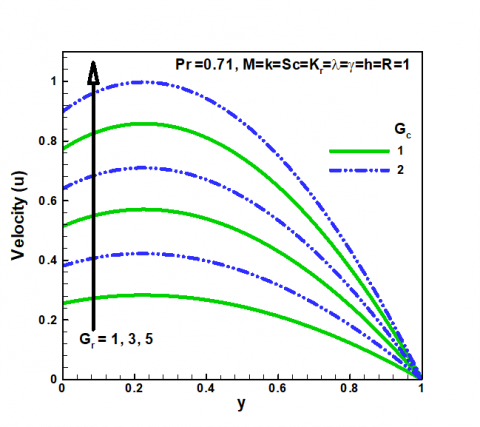Figure 2. Effects of $G c, G_{r}$on velocity

## figure_3.png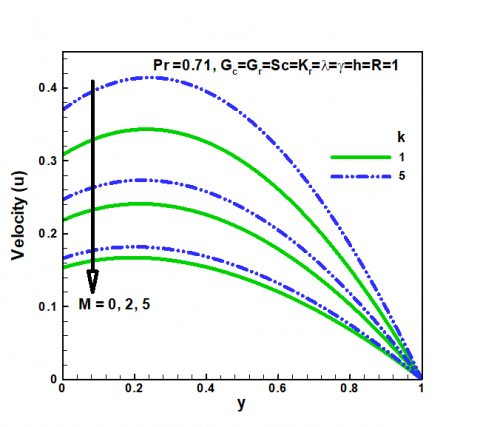Figure 3. Effects of $M, k$ on velocity

## figure_4.png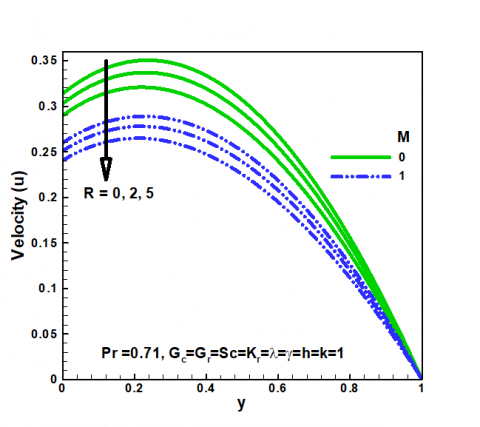Figure 4. Effects of $R, M$on velocity

## figure_5.png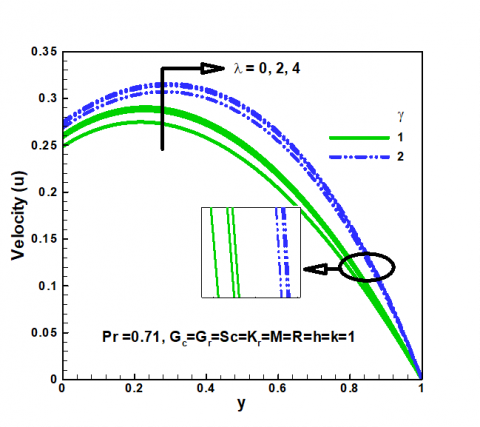Figure 5. Effects of $\lambda, \gamma$ on velocity

## figure_6.png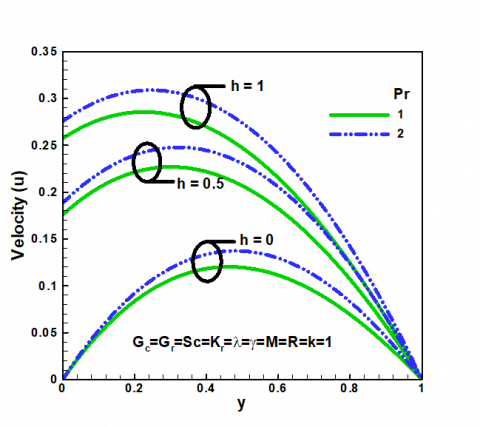Figure 6. Effects of $\operatorname{Pr}, h$ on velocity

## figure_7.png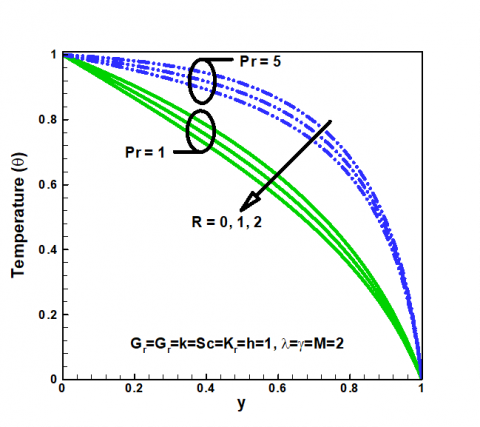Figure 7. Effects of $\operatorname{Pr}, R$ on temperature

## figure_8.png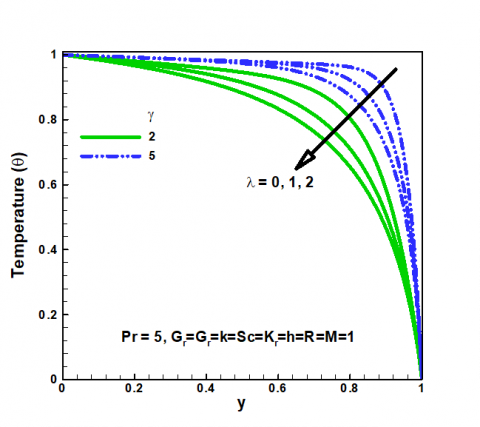Figure 8. Effects of $\lambda, \gamma$ on concentration

## figure_9.png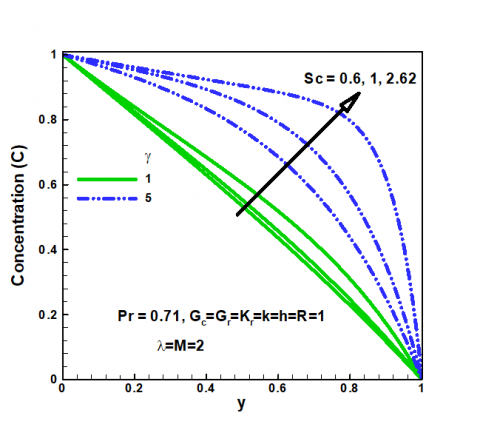Figure 9. Effects of $S c, \gamma$ on concentration

## figure_10.png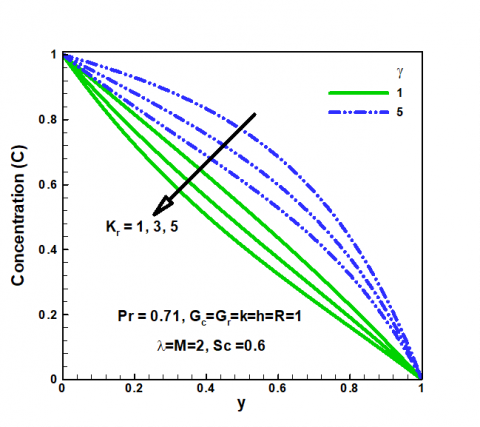Figure 10. Effects of $K_{r}, \gamma$ on concentration

## figure_11.png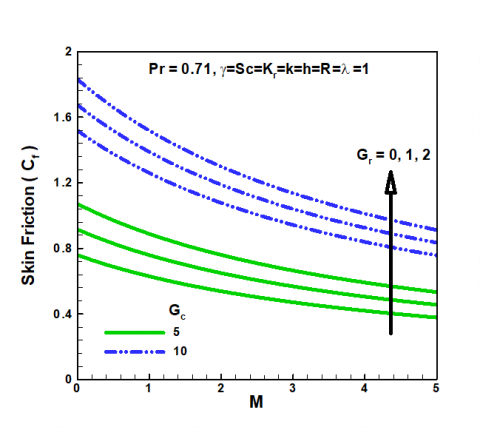Figure 11. Effects of $M, G_{c}$ and $G_{r}$ on skin friction

## figure_12.png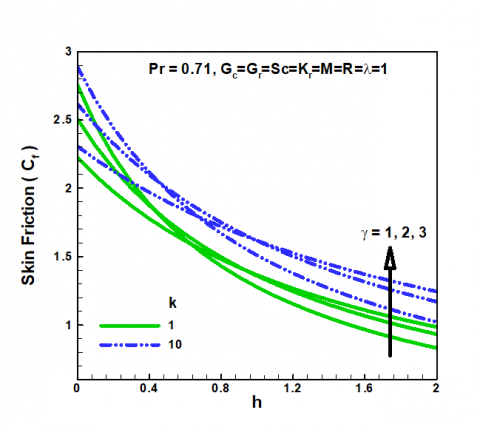Figure 12. Effects of $k, h$ and $\gamma$ on skin friction

## figure_13.png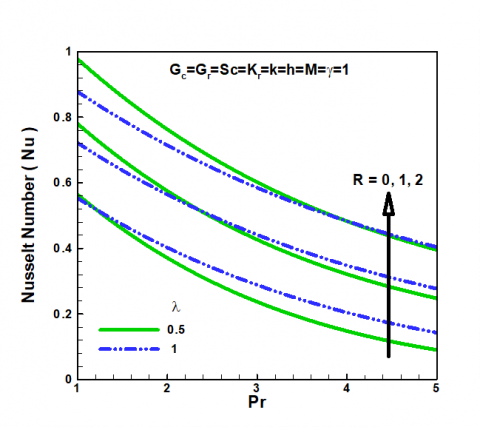Figure 13. Effects of Pr, $\lambda$ and $R$ on Nusselt number

## figure_14.png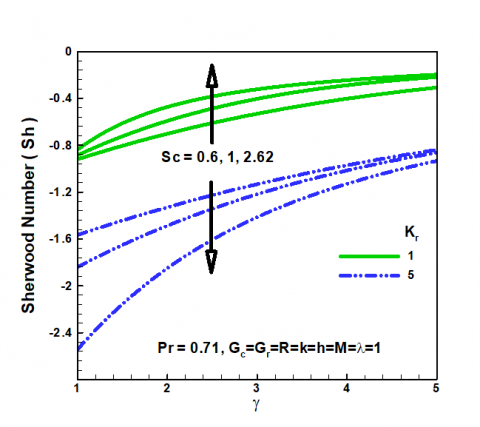Figure 14. Effects of $\gamma, S c$ and $K_{r}$ on Sherwood number

Figure 2 exhibits the effects of Gc and Gr on the velocity profile. It is observed that the velocity profile significantly increased with increasing values of Gc and Gr. As expected, the fluid velocity increases due to the enhancement of thermal and species buoyancy forces. Figure 3 demonstrates the influence of M and k on the velocity profile. It was found that the velocity profile insignificantly reduced with increasing values of M and k. It is interesting to report that application of the transverse magnetic field plays the role of a resistive force (Lorentz force) similar to drag that acts opposite to direction of fluid motion and resist flow thereby reducing its velocity. From the same figure, it is observed that the velocity profile becomes thick for the increase of the permeability parameter k. Similarly, figure 4 described the effect of radiation parameter on the velocity profile. An increase in R leads to decreasing the fluid boundary layer. This is because large values of R correspond to an increased dominance of conduction over R thereby decreasing the buoyancy thickness of the momentum boundary layer. Figure 5 reports the velocity profile for different values of variable thermal conductivity ($\lambda$) and suction parameter ($\gamma$). It I s noticed that the velocity profile increases as a result of increase in any of the parameters.

Figures 6 and 7 depicts the effects of Pr, h and R. It can be observed from the two figures that both the velocity and the temperature profiles insignificantly decreased as a result of increase in the parameters. This is true since at high Prandtl number, the thermal diffusivity of the fluid reduced and causes weak penetration of heat inside the fluid and consequently reduces the velocity of the fluid and the temperature profile. Similarly, high values of radiation parameter causes increased dominance of conduction thereby decreasing the temperature profile.

Figures 8 and 9 indicates that high values of $\lambda$ and $\gamma$ substantially decrease the concentration profile. This is due to the fact that suction parameter decelerates fluid particles through porous wall thereby reducing the species boundary layer growth that results in a decrease in the concentration profile. The values of Sc number are chosen for water (Sc = 0.64), methanol (Sc = 1.0) and propyl benzene (Sc= 2.62). As illustrated physically in figure 8, the outcome of the results shows that high value of Schmidt number increases the concentration boundary thickness thus increasing the concentration gradient at the wall.

Figure 10 reveals the concentration profile for different values of $K_{r}$. It is noticed that with increase in the chemical reaction parameter, the concentration profile decreased. This is evident that an increase in chemical reaction parameter significantly alters the concentration boundary layer thickness and thereby reducing the concentration profile.

In figures 11 and 12, the combined influence of M, Gc and Gr on the local skin friction is observed in the presence of $\operatorname{Pr}, K_{r}, \lambda, \gamma, h$ and k. We found a monotonic increase in the skin friction coefficient when Gc, Gr, k and $\gamma$ are increased. The values of Pr, $\lambda$ and R on the reduced Nusselt number are depicted in figure 13 for fixed values of Gc, Gr, Sc, M, h, k and $\gamma$. We noticed that the rate of heat transfer increases with increasing in all parameters. Figure 14 addresses the influence of $\gamma$, Sc and $K_{r}$ on Sherwood number. It can be seen that the Sherwood number increases and decreases slightly with Schmidt number and Prandtl number respectively.

5. Conclusions

The theoretical investigation of heat and mass transfer with radiation effect and slip condition has been studied. The governing equations are solved numerically by employing an efficient numerical scheme of Runge-Kutta Fehlberg fifth (RKF-45) order. The effects of governing parameters on the velocity flow, temperature, concentration, skin friction, rate of heat and mass transfer characteristics are presented graphically and quantitatively.

We conclude from these results that:

• The velocity profile enhances for the increase in the values of Gc, Gr, $\lambda$ and $\gamma$ while the contrast trend is found on M, k, R, h and Pr.
• The temperature profile decreases with increase in Pr and R.
• The concentration profile decreases with increase in,$\lambda, \gamma$ and $K_{r}$ while accelerates for an increase in Sc.

High values of M, Gc, Gr, k, h and $\gamma$ appreciate skin friction. However, the Prandtl number, radiation and variable thermal conductivity influences the values of the rate of heat and mass transfer.

It is presumed that, with the help of our present model, the physics of flow along vertical channel can be utilized as the basis for many engineering and scientific applications. The findings of the present problem are also of great interest in geophysics particularly in certain geothermal areas. Also, the study finds applications in high-temperature manufacturing processes which are more important in complex polymers.

Nomenclature
 C Concentration $C_{f}$ skin friction $M$ magnetic field parameter g $C_{p}$ gravitational acceleration, m.s-2 specific heat, J. kg-1. K-1 D mass diffusivity $G r$ Grashof number $G c$ solutal Grashof number Nu Pr $S_{\mathcal{C}}$ k $R$ $K_{r}$ local Nusselt number along the heat Prandtl number Schmidt number porous parameter radiation parameter chemical reaction parameter T Sh u, v x, y temperature Sherwood number velocities in the x and y-direction cartesian coordinates along the plate $B_{0}$ magnetic field of constant strength Greek symbols $\beta^{*}$ coefficient of expansion with concentration $\beta$ thermal expansion coefficient, K-1 $\sigma_{0}^{\prime}$ Stefan-Boltzmann constant $\sigma$ scalar electrical conductivity Ρ θ υ $\gamma$ $\lambda$ density of fluid dimensionless temperature kinematic viscosity suction parameter  thermal conductivity, W.m-1. K-1 Subscripts W condition at wall
References

 H. I. Anderson. (2002). Slip flow past a stretching surface.       Acta Mechanica, [Online]. 158,  pp. 121-125. Available: springer.com/article/10.1007%2FBF01463174.pdf

 M. Aarti, M. G. Gorla.  Effect of hall current on unsteady MHD flow of viscoelastic fluid with slip condition and thermal diffusion past an infinite-vertical porous channel through porous medium, Int. J. Phys. Math. Sci., Vol. 4, pp. 349-358, (2013).

 A. Aziz, Hydrodynamic and thermal slip flow boundary layer over a flat plate with constant heat flux boundary conditions, Commun. in Non-Linear Sci. Numer.Simul., Vol.15, pp. 573-580, 2010. DOI:10.1016/j.cnsns.2009.04.026.

 B. Bhattacharyya, S. Mukhopadhyay, G. C. Layek. (2010). Slip Effects on Boundary Layer Stagnation Point Flow and Heat Transfer Towards a Shrinking Sheet, Int. J. Heat Mass Transf., [Online]. 54, pp. 308-313. Available: www.sciencedirect.com/sciencejournal/00179310/54/

 M. Q. Brewster. Thermal Radiative Transfer and Properties, John Wiley and Sons. Inc., New York, 1992.

 A. J. Chamkha. Coupled Heat and Mass Transfer by Natural Convection about a Truncated Cone in the Presence of Magnetic Field and Radiation Effects, Numerical Heat Transfer A, Vol. 39, pp. 511-530, 2001. DOI: 10.1080/10407780120202.

 K. Chand, R. Kumar, S. Sharma. (2012). Combined Effects Of Slip and Jump Boundary Conditions on Oscillatory MHD Flow of Viscoelastic Fluid Considering Soret Effect, Int. J. Phys. Math. Sci. [Online]. 3, pp. 37-44. Available: cuhimakhal.ac.in/news/Profiles/20130513165349587.pdf

 J. A. Gbadeyan, M. S. Dada. On the Influence of Radiation and Heat Transfer on an Unsteady MHD Non-Newtonian Fluid Flow with Slip in a Porous Medium, J. Math.  Res, Vol.5, pp. 40-50, 2013. DOI: 105539/jmr.v5n3p40.

 Goldstein. (1965). Modern Developments in Fluid Dynamics, [Online]. 2, pp. 676. Available: www.ijemail.org/reference/Referencespapers.aspx?

 T. Hayat, M. Qasim, Mesloub. MHD Flow and Heat Transfer over Permeable Stretching Sheet with Slip Conditions, Int.  J. Numer.Methods in Fluids, Vol.66, pp. 963-975, 2010. DOI: 10:1002/fld.2294.

 M. A. Hossain, M. A. Alim, D. A. S. Rees. (1999). Effect of Radiation on Free Convection from a Porous Vertical Plate, Int. Heat Mass Transf., [Online]. 42, pp. 181-191. Availabe: https//books.goggle.com.ng/books?

 D. B. Ingham, I. Pop. Transport Phenomena in Porous Media. Elsevier, Oxford, UK. 2005.

 P. M. Kishore, N. V. Prasada, S. V. Varma, S. Vantarakamana. (2013). The Effects of Radiation and Chemical Reaction on Unsteady MHD Free Convection Flow of Viscous Fluid Past an Exponentially Accelerated Vertical Plate, Int. J. Phys. Math. Sci., [Online]. 4, pp. 300-317. Available: https://www.researchgate.net/publication

 B. Krishnendu. Slip Effects on Boundary Layer Flow     And Mass Transfer with Chemical Reaction over a Permeable Flat Plate Embedded in a Darcy Porous Medium, Frontiers Heat and Mass Transf., Vol. 3, pp. 1-6, 2012. DOI: 10.5098/hmt.v3.4.3006.

 G. Murali, N. V. N. Babu. (2012).  Effect of Radiation on MHD Convection Flow Past a Vertical Permeable Moving Plate, Int. J. Adv. Appl. Sci., [Online]. 1, pp.19-28. Available: https://iaesjournal.com/online/index.php/IJAAS.

 D. A. Nield, A. Bejan. Convection in Porous Media, third edition, Springer, New York, 1998.

 S. Nityananda, K. D. Rajendra. (2013). Effect of Slip Condition on Unsteady MHD Oscillatory Flo w in a Channel Filled with Porous Medium with Heat Radiation and Mass Transfer, Int. J. Appl. Math. Statist. Sci., [Online]. 2, pp. 11-20. Available: http.journalajst.com/sites/default/files/1536.pdf

 U. R. Rajeshwara, V. P. Ramachandra, G. Viswanath,  B. Vasu. (2012). Radiation Effects on Unsteady Free Convection Heat And Mass Transfer in a Walters-B Viscoelastic Flow Past an Impulsively Started Vertical Plate, Int. J. Sci. Eng.  Res., [Online]. 3, pp. 1-9. Available: http://www.ijear.org

 U. S. Rajput, S. Kumar. (2012). Radiation Effects on MHD Flow Past an Impulsively Started Vertical Plate with Variable Heat and Mass Transfer, J. Appl. Math. Mech., [Online]. 8, pp. 66-85. Available: https://www.sciencedirect.com/science

 V. M. Soundalgekhar, H. S. Takher. (1992). Radioactive Convective Flow Past a Semi-Infinite Vertical Plate, Modeling Measure and Control., [Online]. 51, pp. 31-40. Available: Shodhganga.inflibnet.ac.in/bitstream/10603/28659/11/11-refrences.pdf

 S. Suneetha, N. R. Bhaskhar. (2011). Radiation and Darcy Effects on Unsteady MHD Heat and Mass Transfer Flow of a Chemically Reacting Fluid Past an Impulsively Started Vertical Plate with Heat Generation, Int. J. Appl. Math. Mech. [Online]. 7, pp. 1-19. Available: studylib.net/abstracts.apsms.org

 H. S. Takher, S. R. Garla, V. M. Soundalgekhar. (1996). Radiation Effects on MHD Free Convection Flow of a Radiating Gas Past a Semi-Infinite Vertical Plate, Int. J. Numer. Meth. Heat Fluid Flow, [Online]. 6, pp. 77-83. Available: http://dx.doi.org/10.1108/09615539610113118

 Z. Uddin, K. Manoj. (2010). Radiation Effect on Unsteady MHD Heat and Mass Transfer Flow on a Moving Inclined Porous Heated Plate in the Presence of Chemical Reaction, Int. J. Math. Mod., Simul. Appl. [Online]. 3, pp. 155-163. Available: www.academia.edu

 N. Ahmed, K. D. Kishore. MHD mass transfer flow past a vertical porous plate embedded in a porous medium in a slip flow regime with thermal radiation and chemical reaction, Open  J. Of Fluid Dynamics, [Online]. 3(3), pp. 230-239, (2013). DOI: 10.4236/ojfd.2013.33028.

 P. Loganathan, C. Sivapoornapriya. (2014). Unsteady natural convection flow of a micropolar fluid past a vertical moving porous plate in the presence of porous medium with radiation and chemical reaction, Int. J. Heat and Technology, [Online]. 33(1&2), pp. 87-94. Available: https://www.researchgate.net/publication/288217645

 S. I. Mohammed, K. Suneetha. (2016). Effects of thermal diffusion and chemical reaction on MHD transient free convection flow past a porous vertical plate with radiation, temperature gradient heat source in slip flow regime, Winter and Spring,  [Online]. 5(2), pp. 83-95. Available: http://jcarme.srttu.edu